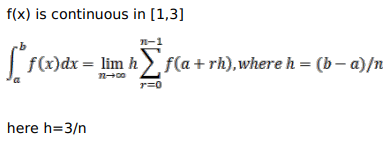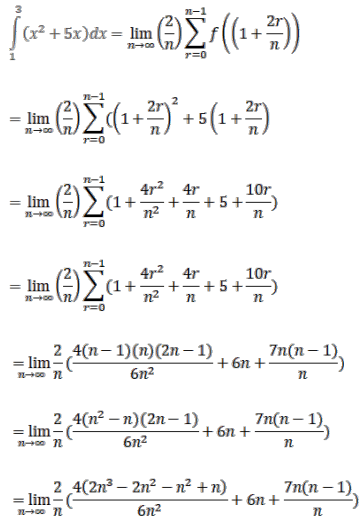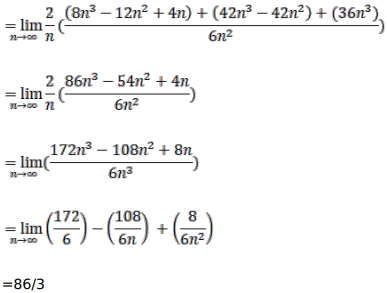# Evaluate each of the following integrals as the limit of sums:Question:

Evaluate each of the following integrals as the limit of sums:

$\int_{1}^{3}\left(x^{2}+5 x\right) d x$

Solution: